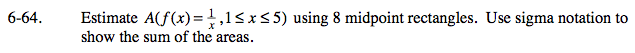### Home > PC > Chapter 6 > Lesson 6.1.4 > Problem6-64

6-64.Determine the width of each rectangle.

$\frac{\text{length of interval}}{\text{number of rectangles}}$

Find the midpoint for each rectangle.

x0 = 1.25, x1 = 1.75, ...

Use sigma notation to show the sum of the areas of the 8 rectangles.

$\text{width}\sum_{n=0}^{7}\frac{1}{\text{(expression for }x_n\text{s})}$